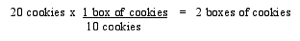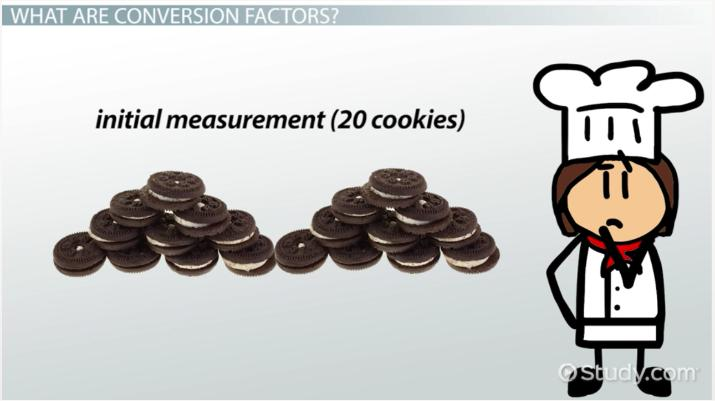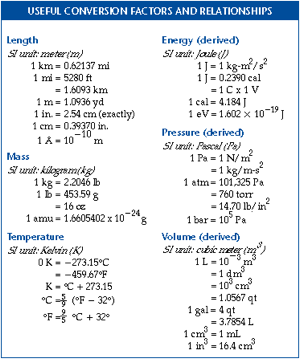Science Courses / Course / Chapter

# Conversion Factor in Chemistry: Definition, Formula & Practice Problems

Lesson Transcript
Instructor: Sheila Morrissey

Sheila has a master's degree in geology and has taught middle school through university-level science courses.

In chemistry, conversion factors are used to convert units into the unit appropriate for the situation, such as changing metric units to English units. Explore the definition of conversion factors in chemistry, review conversion formulas, understand how to set up unit conversions, and learn more by doing practice problems. Updated: 09/21/2021

## What Are Conversion Factors?

If you have 20 cookies and you multiply that by 1, you still have the same amount of cookies. You might, however, wonder how many boxes of cookies that equals. To figure it out, you will need a conversion factor, a ratio expressed as a fraction that equals 1. It will allow you to change units while maintaining your original measurements.

So in the case of the cookies, we need to think about our initial measurement (20 cookies) and the final units in which we want to be able to express that amount (boxes of cookies). We don't want to give away any of those cookies or eat them or accept more; we simply want to convert our expression from 20 cookies to the same amount expressed in boxes of cookies. So we need to measure or look up a conversion factor.We started with 20 cookies, and we have a conversion factor of 10 cookies per box. Our initial units in this case are cookies, and the final units we want are boxes. In order to cancel out the cookies units and introduce the boxes units, we will need to place the cookies units in the denominator of the conversion factor fraction and keep cookie box units in the numerator. We multiply 20 cookies by our conversion factor and find that the same measurement of cookies can also be expressed as 2 boxes of cookies.An error occurred trying to load this video.

Try refreshing the page, or contact customer support.

Coming up next: Density: Definition, Formula & Practice Problems

### You're on a roll. Keep up the good work!

Replay
Your next lesson will play in 10 seconds
• 0:01 What Are Conversion Factors?
• 1:48 Examples of Conversion Factors
• 2:56 Setting Up Unit Conversions
• 4:16 Practice Problems
• 6:10 Lesson Summary
Save Timeline
Autoplay
Autoplay
Speed Speed

## Examples of Conversion Factors

In chemistry, we aren't likely to encounter cookie problems often. We will, instead, use conversion factors to change between English and metric units, to quantify moles or atoms, or to use bigger or smaller units within a unit system. Most often, we'll be converting between like units, so we'll convert from one unit of time to another unit of time, or from one unit of mass to another unit of mass, for example. Or maybe, we'll just quantify a number of atoms and put it into moles. It is also possible to convert between dimensions with a known conversion factor, such as 1 kilogram of pure water equals 1 liter.

You might already know some conversion factors without having to look them up, like 12 inches per foot, 60 seconds per minute, 60 minutes per hour, and 24 hours per day. If you will be doing a lot of molar mass calculations, for instance, you might want to memorize the definition of a mole, 6.022 x 10^23. A mole is just a number, the way a dozen means 12. It is easy to look up common conversion factors though, too, in a table like this.## Setting Up Unit Conversions

We have described conversion factors as fractions equaling 1. When we use conversion factors to convert units, we will multiply our original measurement by the conversion factor to get the same measurements expressed in new units. We need to be careful in setting up our multiplication problem correctly so that we cancel out our old units and introduce the new units in the numerator of the conversion factor fraction.

Using the table above, we see that 1 kilometer is equal to approximately 0.62137 miles. If we want to convert 5.0 miles to kilometers, we multiply our original measurement (5.0 miles) by the conversion factor. If we multiplied it by (0.62137 miles/1 kilometer), which is, indeed, a fraction equaling 1, we would end up with final units of miles squared divided by kilometers. Those units are not what the problem called for and don't make sense as a unit of length. So it's important that we look ahead to the final units we are looking for. In this case, those units are kilometers.

To unlock this lesson you must be a Study.com Member.

### Register to view this lesson

Are you a student or a teacher?

Back

### Resources created by teachers for teachers

Over 30,000 video lessons & teaching resources‐all in one place.Video lessonsQuizzes & WorksheetsClassroom IntegrationLesson Plans

I would definitely recommend Study.com to my colleagues. It’s like a teacher waved a magic wand and did the work for me. I feel like it’s a lifeline.

Jennifer B.
TeacherCreate an account to start this course today
Used by over 30 million students worldwide